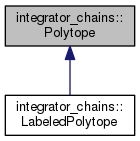fmrbenchmark  0.0.4
integrator_chains::Polytope Class Reference

Basic half-space representation of polytopes. More...

`#include <polytope.hpp>`

Inheritance diagram for integrator_chains::Polytope:[legend]

## Public Member Functions

void dumpJSONcore (std::ostream &out) const
Output this polytope in JSON excluding opening { and closing }. More...

bool is_consistent () const
Check that defining matrices have consistent dimensions. More...

bool is_in (Eigen::VectorXd X) const
Is X contained in this polytope? More...

Polytope operator& (const Polytope &P2)
Intersect two polytopes that are defined in the same dimension space. More...

Polytope (Eigen::MatrixXd incoming_H, Eigen::VectorXd incoming_K)

Polytope (const Polytope &other)

## Static Public Member Functions

static Polytopebox (const Eigen::VectorXd &bounds)
Construct Polytope that is an axis-aligned rectangle. More...

static PolytoperandomH (int n)
Create random Polytope in R^n using Eigen::MatrixXd::Random(). More...

## Friends

std::ostream & operator<< (std::ostream &out, const Polytope &P)
Output this polytope in JSON to given stream. More...

## Detailed Description

Basic half-space representation of polytopes.

## Member Function Documentation

 Polytope * integrator_chains::Polytope::box ( const Eigen::VectorXd & bounds )
static

Construct Polytope that is an axis-aligned rectangle.

Parameters
 bounds an array of the form [x1_min, x1_max, x2_min, x2_max, ..., xn_min, xn_max], which defines a polytope in terms of intervals along each axis in R^n. The interval along the first axis is [x1_min, x1_max], the interval along the second axis is [x2_min, x2_max], etc. Thus the length of the given array is 2n.

E.g., a unit square in R^2 can be created using

Eigen::Vector4d bounds;
bounds << 0, 1,
0, 1;
Polytope *square = Polytope::box( bounds );
 void integrator_chains::Polytope::dumpJSONcore ( std::ostream & out ) const

Output this polytope in JSON excluding opening { and closing }.

This method facilitates inheritance from Polytope without crowding the JSON representations of other classes.

 bool integrator_chains::Polytope::is_consistent ( ) const

Check that defining matrices have consistent dimensions.

The "defining matrices" are those involved in the inequality providing the half-space representation, i.e., H and K in {x | Hx <= K}. N.B., this check does not occur during instantiation of Polytope.

 bool integrator_chains::Polytope::is_in ( Eigen::VectorXd X ) const

Is X contained in this polytope?

The polytope is a closed set. No numerical tolerance is used.

 Polytope integrator_chains::Polytope::operator& ( const Polytope & P2 )

Intersect two polytopes that are defined in the same dimension space.

 Polytope * integrator_chains::Polytope::randomH ( int n )
static

Create random Polytope in R^n using Eigen::MatrixXd::Random().

Parameters
 n dimension of the containing space.

Random matrices as provided by Eigen::MatrixXd::Random() are used to instantiate Polytope in half-space representation. The matrices have `n+1` rows, corresponding to `n+1` inequalities.

## Friends And Related Function Documentation

 std::ostream& operator<< ( std::ostream & out, const Polytope & P )
friend

Output this polytope in JSON to given stream.

The documentation for this class was generated from the following file:
• polytope.hpp